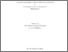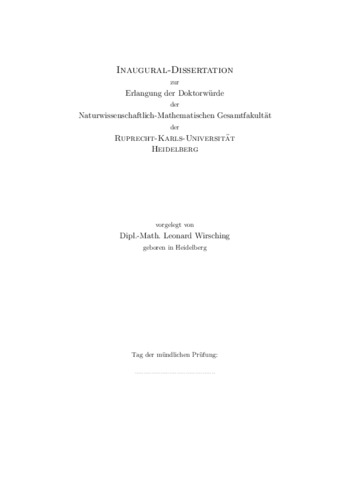## Multi-Level Iteration Schemes with Adaptive Level Choice for Nonlinear Model Predictive Control

Wirsching, LeonardPreview
PDF, English

Citation of documents: Please do not cite the URL that is displayed in your browser location input, instead use the DOI, URN or the persistent URL below, as we can guarantee their long-time accessibility.

## Abstract

In this thesis we develop the Multi-Level Iteration schemes (MLI), a numerical method for Nonlinear Model Predictive Control (NMPC) where the dynamical models are described by ordinary differential equations. The method is based on Direct Multiple Shooting for the discretization of the optimal control problems to be solved in each sample. The arising parametric nonlinear problems are solved approximately by setting up a generalized tangential predictor in a preparation phase. This generalized tangential predictor is given by a quadratic program (QP), which implicitly defines a piecewise affine linear feedback law. The feedback law is then evaluated in a feedback phase by solving the QP for the current state estimate as soon as it becomes known to the controller.

The method developed in this thesis yields significant computational savings by updating the matrix and vector data of the tangential predictor in a hierarchy of four levels. The lowest level performs no updates and just calculates the feedback for a new initial state estimate. The second level updates the QP constraint functions and approximates the QP gradient. The third level updates the QP constraint functions and calculates the exact QP gradient. The fourth level evaluates all matrix and vector data of the QP. Feedback schemes are then assembled by choosing a level for each sample. This yields a successive update of the piecewise affine linear feedback law that is implicitly defined by the generalized tangential predictor.

We present and discuss four strategies for data communication between the levels in a scheme and we describe how schemes with fixed level choices can be assembled in practice. We give local convergence theory for each level type holding its own set of primal-dual variables for fixed initial values, and discuss existing convergence theory for the case of a closed-loop process. We outline a modification of the levels that yields additional computational savings.

For the adaptive choice of the levels at runtime, we develop two contraction-based criteria to decide whether the currently used linearization remains valid and use them in an algorithm to decide which level to employ for the next sample. Furthermore, we propose a criterion applicable to online estimation. The criterion provides additional information for the level decision for the next sample. Focusing on the second lowest level, we propose an efficient algorithm for suboptimal NMPC.

For the presented algorithmic approaches, we describe structure exploitation in the form of tailored condensing, outline the Online Active Set Strategy as an efficient way to solve the quadratic subproblems and extend the method to linear least-squares problems. We develop iterative matrix-free methods for one contraction-based criterion, which estimates the spectral radius of the iteration matrix.

We describe three application fields where MLI provides significant computational savings compared to state-of-the-art numerical methods for NMPC. For both fixed and adaptive MLI schemes, we carry out extensive numerical testings for challenging nonlinear test problems and compare the performance of MLI to a state-of-the-art numerical method for NMPC. The schemes obtained by adaptive MLI are computationally much cheaper while showing comparable performance. By construction, the adaptive MLI allows giving feedback with a much higher frequency, which significantly improves controller performance for the considered test problems.

To perform the numerical experiments, we have implemented the proposed method within a MATLAB(R) based software called MLI, which makes use of a software package for the automatic derivative generation of first and higher order for the solution of the dynamic model as well as objective and constraint functions, which performs structure exploitation by condensing, and which efficiently solves the parametric quadratic subproblems by using a software package that provides an implementation of the Online Active Set Strategy.

Item Type: Dissertation Bock, Prof. Dr. Dr. h.c. mult. Hans Georg 24 April 2018 14 May 2018 06:51 2018 The Faculty of Mathematics and Computer Science > Dean's Office of The Faculty of Mathematics and Computer ScienceService facilities > Interdisciplinary Center for Scientific Computing 510 Mathematics Modellprädiktive Regelung, Zustandsschätzung, Direkte Methode, Mehrzielmethode, Sequenzielle quadratische Optimierung, Parametrische Optimierung, Nichtlineare gewöhnliche Differentialgleichung